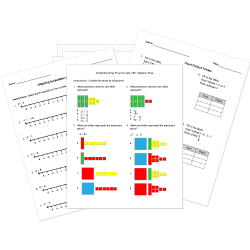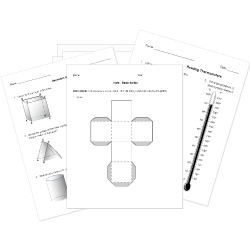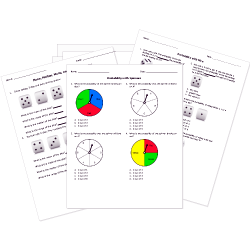Tweet# Printable Math Worksheets, Tests, and Activities

Assess student understanding of arithmetic, algebra, and geometry concepts with these math worksheets for K-12 students.

Worksheets labeled with are accessible to Help Teaching Pro subscribers only. Become a Subscriber to access all standards aligned worksheets.

Related Articles: 11 Prime Days to Celebrate Math Holidays and 9 Ways to Make Math Relevant

## PARCC Style Practice and Review Worksheets

These tests feature questions modeled after PARCC Mathematics items. Use Test Room to administer these tests for computer-based assessment practice. Search for PARCC questions to see all content available and to create your own custom assessments.

### Arithmetic and Number ConceptsPractice arithmetic skills with this collection of math worksheets for addition, subtraction, multiplication, division, fractions, and decimals. Answer pictograph questions for basic addition, subtraction, and fraction problems. Develop counting skills, introduce inequality signs, complete patterns, and solve problems with negative numbers with these printable math sheets.

All arithmetic and number concepts worksheets »

### Function and Algebra ConceptsFunction and Algebra Concepts Use these Pre-Algebra, Algebra I, and Algebra II tests to gauge student comprehension of algebra topics including: linear and quadratic equations, inequalities featuring number line graphics, functions, exponents, radicals, and logarithms.

All function and algebra concepts worksheets »

## Geometry and MeasurementInvestigate lines, angles, circles, triangles, polygons, perimeter, volume, and the coordinate plane, among other topics with these geometry worksheets. Print net activities (3D models) for hands-on student learning. Learn about measurement using these sheets featuring pictures of coins, clocks, and thermometers. Math tests include converting customary and metric units within a measurement system.

All geometry and measurement worksheets »

### Statistics and Probability ConceptsThe ability to estimate, interpret, and manipulate data is an essential skill. Strengthen student understanding of probability and statistics concepts with these printables.

All statistics and probability worksheets »

### Trigonometry

Explore topics such as trigonometric ratios and functions, Sine and Cosine Law, trigonometric identities, the unit circle, and radians and degrees with these worksheets.

All trigonometry worksheets »

### Pi Day (March 14) Math Worksheets

Celebrate Pi Day with this collection of worksheets - print or administer online tests with Test Room.